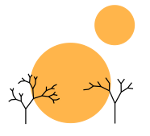# Uniform Computability

Rimu Shuang. "Untitled Photo". Jan 15, 2014. Under a Creative Commons 3.0 Attribution License.

### computability_theory, math

I’ve been doing a bunch of reading for school and invariably the words “uniform computability” pop up a lot, but to the best of my knowledge, I don’t think I’ve actually seen a place where it’s actually been defined. I’ve yet to see where the notion of uniform computability is defined, so I’ll take a stab at it here.

Let’s take the simplest example, where we have a set 𝒮 of sets Si. Then say that each set Si is computable and has a computable indicator function $_{S_i}$, although each of these sets is nice and computable, it may be an actual pain to come up with the φSi for each set. It does us very little good to say that all of these sets Si are computable, if we can’t find the computable function associated with each of them.

However, in some circumstances, we can and to describe those circumstances, we use the term “uniform computability.” Specifically 𝒮 is uniformly computable if the Si can be computably enumerated and there is a computable function ψ such that if given i as an input (where i is assumed to be the computable index), it can return the Gödel number of the computable program associated with the indicator function for Si.

More generally, given some computably enumerable set 𝒮 whose elements are each computable, if there is a computable function that yields the Gödel number of the computable program associated with that element, then 𝒮 is uniformly computable.